## MECHANISM INVESTIGATION OF WATER INJECTION ON SUPPRESSING HYDROFOIL CLOUD CAVITATION FLOW 1)

Wang Wei,2), Zhang Qingdian, Tang Tao, An Zhaoyang, Tong Tianhao, Wang Xiaofang

School of Energy and Power Engineering,Key Laboratory of Ocean Energy Utilization and Energy Conservation of Ministry of Education,Dalian University of Technology, Dalian 116024,Liaoning,China

 基金资助: 1) 国家自然科学基金.  51876022国家重点基础研究发展计划.  2015CB057301

Received: 2019-10-14   Accepted: 2019-12-8   Online: 2020-01-18Abstract

In order to understand the development mechanism of cloud cavitation and investigate the influence of jet flow on the suction surface of hydrofoil, the unsteady evolution process of cloud cavitation of the original NACA66(mod) hydrofoil and the hydrofoil with jet flow is simulated and compared by using density modified RNG $k$-$\varepsilon$ turbulence model and Schnerr-Sauer cavitation model. Monitoring lines of the hydrofoil suction surface are adopted and then spatiotemporal evolution of the vapor phase volume fraction, re-entrant jet flow, pressure and pressure gradient near the hydrofoil wall is obtained. The flow field characteristics of the cloud cavitation are analyzed by vortex dynamic. The suppression mechanism of active jet is analyzed. The results show that the first retraction of the attached cavity is caused by the strong local high pressure when the dissociated cavity collapse downstream, and the second retraction is affected by the re-entrant jet. The re-entrant jet zone is limited to higher pressure gradient. High pressure gradient always exists, but the initial occurrence of re-entrant jet in a time period needs the accumulation of time. Jet flow on the suction surface of hydrofoil can reduce cloud cavitation. The suppression mechanism is that injected jet makes the pressure rise near jet holes, making up for the pressure drop caused by cavitation and circumfluence, so as to increase the pressure gradient and enhance the anti-pressure ability. On the other hand, the re-entrant jet strength is decreased. The vortex structure contour of Q criterion shows complex flow structure compared to the vapor phase volume fraction contour. Vortex cores are existed in the front attached cavity and dissociated cavity, while shear may be present in re-entrant jet zone. The shear function of the injected jet suppresses the development of cavitation and re-entrant jet.

Keywords： cavitation suppression ; hydrofoil ; re-entrant jet ; cloud cavitation ; vortex structure

Wang Wei, Zhang Qingdian, Tang Tao, An Zhaoyang, Tong Tianhao, Wang Xiaofang. MECHANISM INVESTIGATION OF WATER INJECTION ON SUPPRESSING HYDROFOIL CLOUD CAVITATION FLOW 1). Chinese Journal of Theoretical and Applied Mechanics[J], 2020, 52(1): 12-23 DOI:10.6052/0459-1879-19-282

## 1 数值模拟方法

### 1.1 控制方程

$\dfrac{\partial \rho _{\rm m} }{\partial t} + \dfrac{\partial \left( {\rho _{\rm m} u_j } \right)}{\partial x_j } = 0$
$\dfrac{\partial \rho _{\rm m} \mu _i }{\partial t} + \dfrac{\partial (\rho _{\rm m} \mu _i \mu _j )}{\partial x_j } = - \dfrac{\partial p}{\partial x_i } + \dfrac{\partial }{\partial x_j }\left[ {(\mu _{\rm m} + \mu _{\rm t} )(\dfrac{\partial \mu _i }{\partial x_j } + \dfrac{\partial \mu _j }{\partial x_i } - \dfrac{2}{3}\dfrac{\mu }{\partial x_j }\delta _{ij} )} \right]$

$\rho _{\rm m} = \rho _{\rm l} \alpha _{\rm l} + \rho _{\rm v} \alpha _{\rm v}$
$\mu _{\rm m} = \mu _{\rm l} \alpha _{\rm l} + \mu _{\rm v} \alpha _{\rm v}$

### 1.2 空化模型

$\dfrac{\partial }{\partial {\rm t}}(a_{\rm v} \rho _{\rm v} ) + \nabla (a_{\rm v} \rho _{\rm v} {\pmb v} ) = R_{\rm e} + R_{\rm c}$

$R_{\rm e} + R_{\rm c} = \dfrac{\rho _{\rm v} \rho _{\rm l} }{\rho _{\rm m} }\dfrac{{\rm D}\alpha _{\rm v} }{{\rm D}t}$

$\alpha _{\rm v} = \dfrac{4n_{\rm b} \pi R_{\rm B}^3/3 }{1 + 4n_{\rm b} \pi R_{\rm B}^{\rm 3}/3 }$

$\left. \begin{array}R_{\rm e} = \dfrac{\rho _{\rm v} \rho _{\rm l} a_{\rm v} \left( {1 - a_{\rm v} } \right)}{\rho _{\rm m} }\dfrac{3}{R_{\rm B} }\sqrt {\dfrac{2\max (p_{\rm v} - p,0)}{3\rho _l }} \\ R_{\rm c} = - \dfrac{\rho _{\rm v} \rho _{\rm l} a_{\rm v} \left( {1 - a_{\rm v} } \right)}{\rho _{\rm m} }\dfrac{3}{R_{\rm B} }\sqrt {\dfrac{2\max (p - p_{\rm v} ,0)}{3\rho _{\rm l} }} \end{array}\!\!\right\}$

### 1.3 湍流模型

$\mu _{\rm t} = \rho c_\mu \dfrac{k^2}{\varepsilon }$

$\mu _t = f\left( \rho \right)c_\mu \dfrac{k^2}{\varepsilon }$
$f\left( \rho \right) = \rho _{\rm v} + \left( {1 - \alpha _{\rm v} } \right)^n\left({\rho _{\rm l} - \rho _{\rm v} } \right)$

### 图1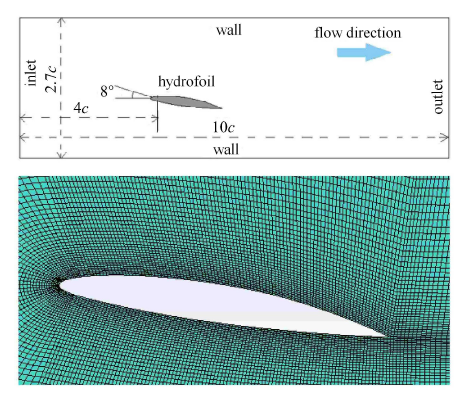Fig. 1   Computational domain and near foil meshing

### 图2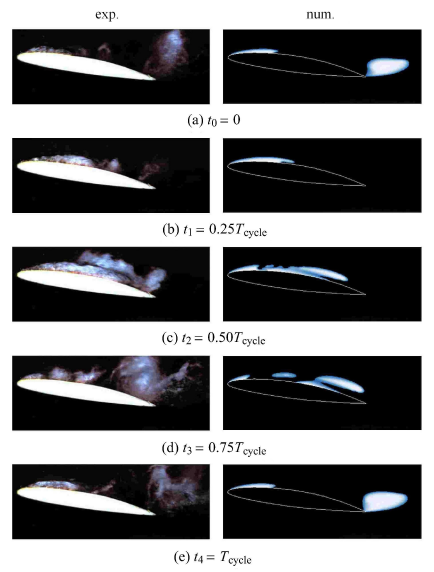Fig. 2   Instantaneous outline of cavities of experiment and simulation in a time period

### 图3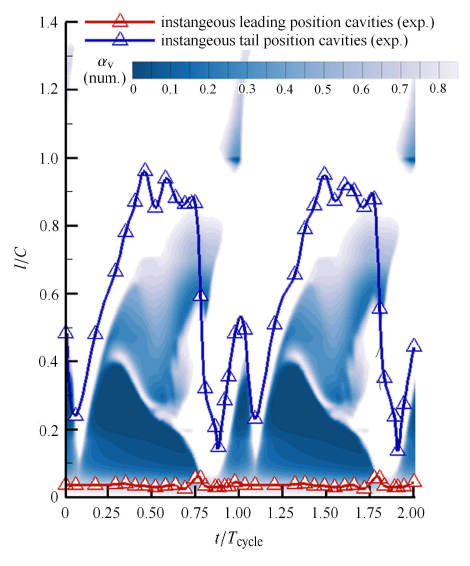Fig. 3   Instantaneous length of cavities of experiment and simulation in two time periods

## 2 结果分析

### 图4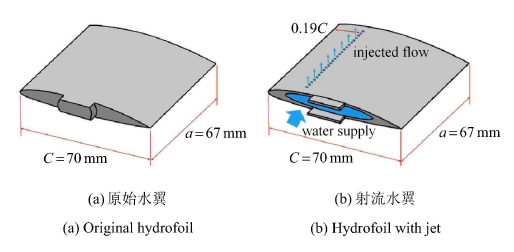Fig. 4   Schematic diagram of hydrofoil

### 图5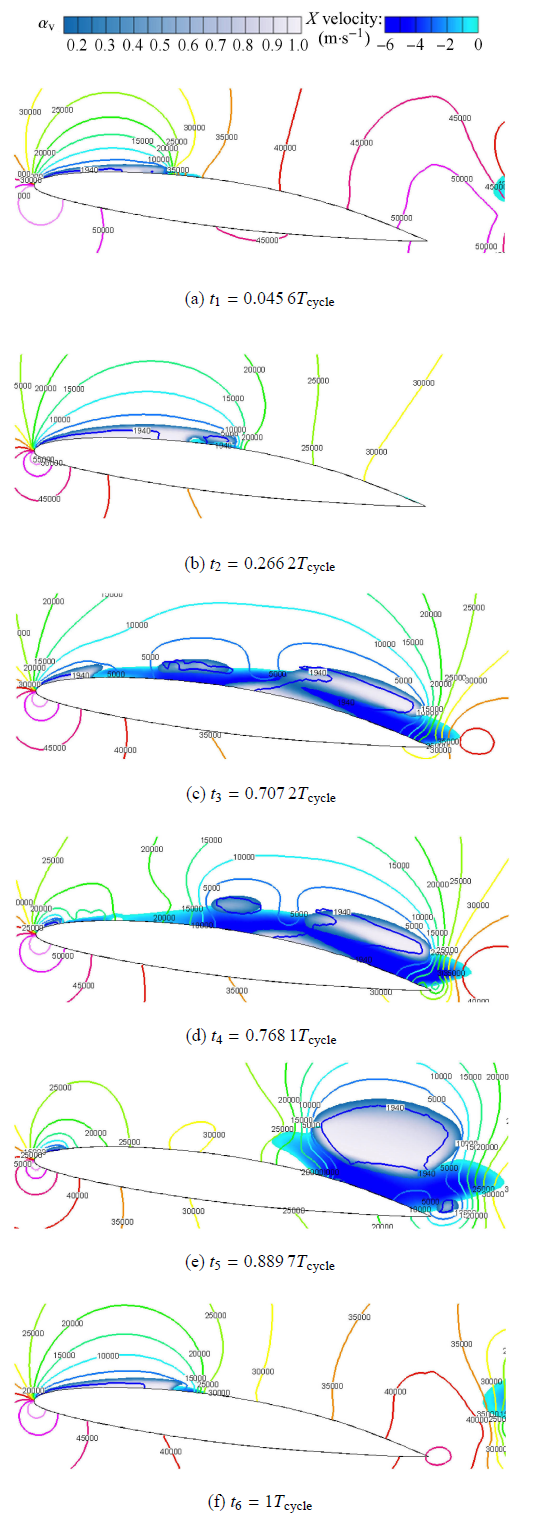Fig. 5   The numerically predicted vapor fraction ($\alpha _{\rm v} >0.15$) of cavitation pattern around a NACA66 hydrofoil ($T_{\rm cycle} = 66$ ms)

### 图6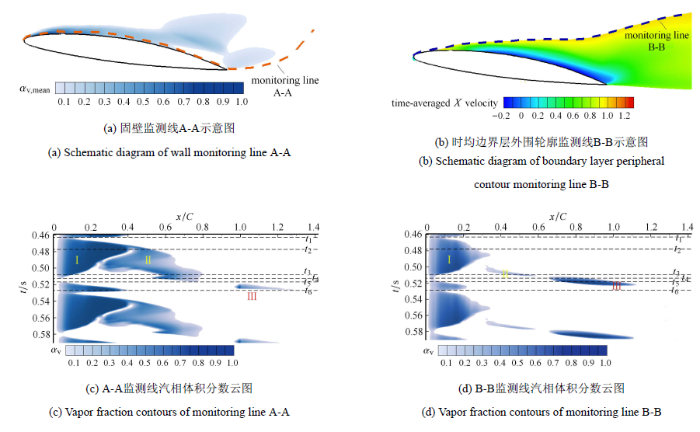Fig. 6   Spatiotemporal distribution of unsteady cloud cavitation flow around NACA66 hydrofoil

### 图6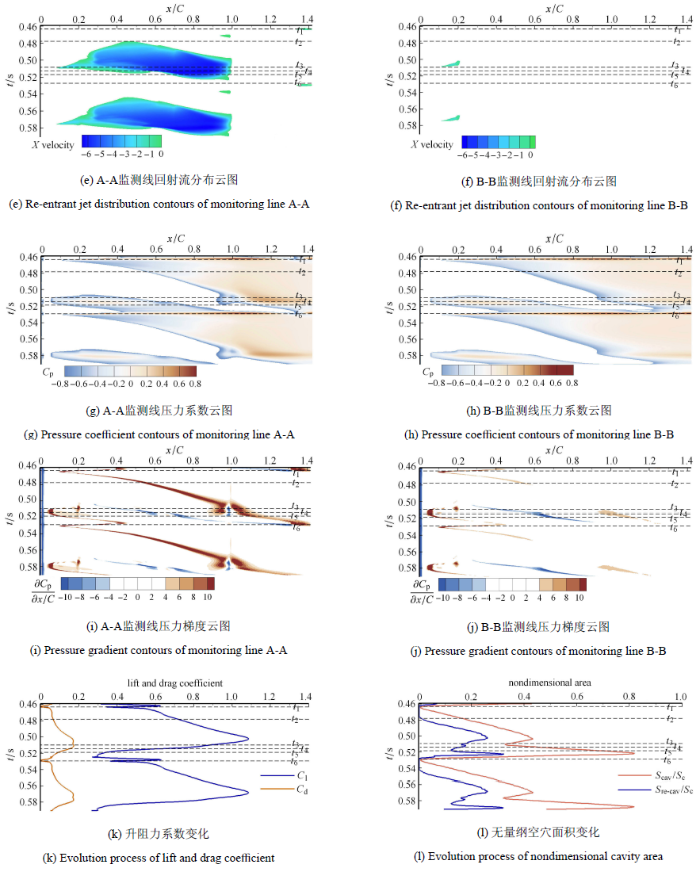Fig. 6   Spatiotemporal distribution of unsteady cloud cavitation flow around NACA66 hydrofoil (continued)

$C_{\rm p} = \dfrac{2(p - p_\infty) }{\rho _{\rm l} U_\infty ^2}$
${\rm grad}C_{\rm p} = \dfrac{\partial C_{\rm p} }{\partial (x/C)}$

### 图7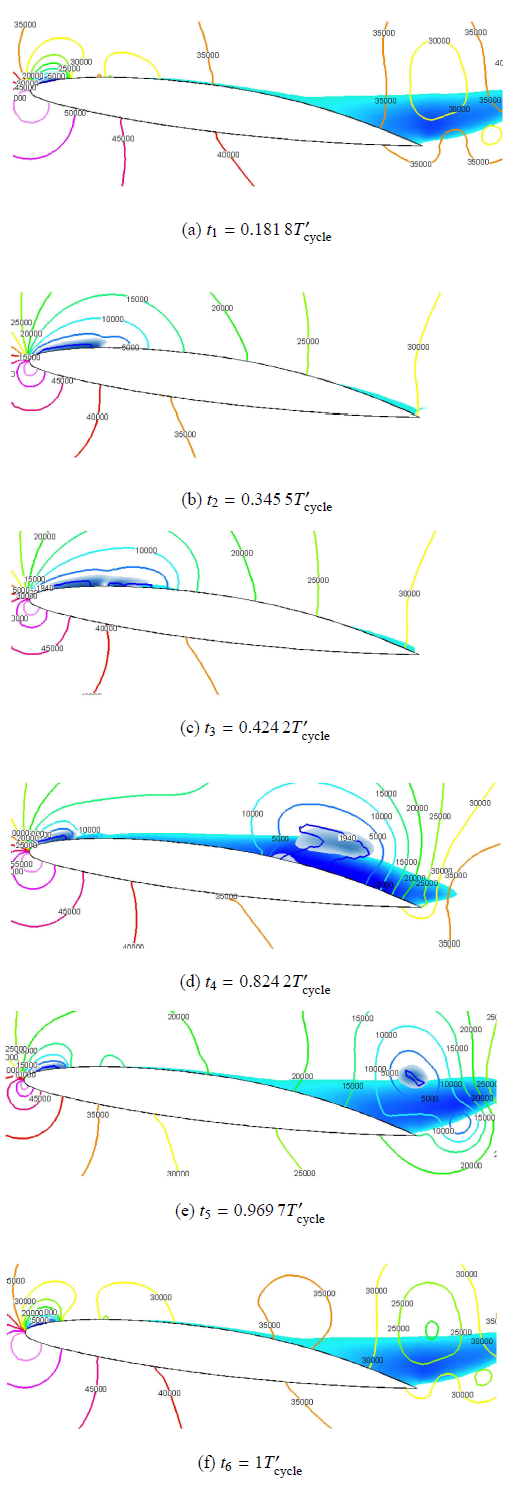Fig. 7   The numerically predicted vapor fraction ($\alpha _{\rm v} >0.15$) of cavitation pattern around a NACA66 hydrofoil with jet flow ($T'_{\rm cycle} = 82.5$ ms)

### 图8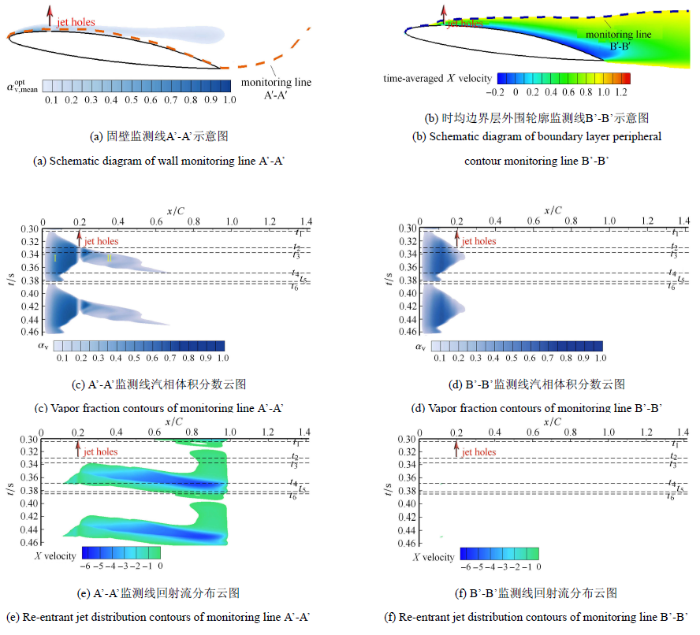Fig. 8   Spatiotemporal distribution of unsteady cloud cavitation flow around NACA66 hydrofoil with jet flow

### 图8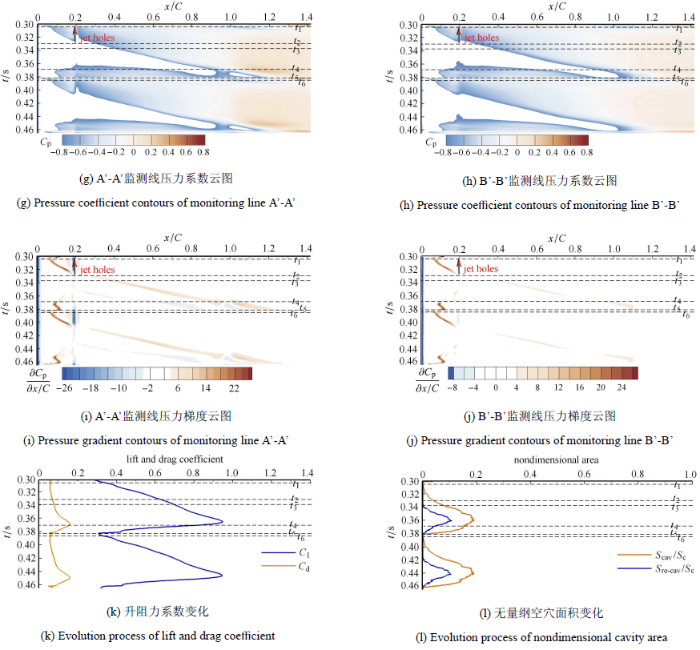Fig. 8   Spatiotemporal distribution of unsteady cloud cavitation flow around NACA66 hydrofoil with jet flow (continued)

### 2.3 空化流动控制的涡旋特性分析

$Q$是在伽利略变换下的速度梯度张量第二不变量,近年来,研究者认为以$Q$显示的涡流能够更清晰的反应流场内的涡旋结构,且空化与旋涡间存在交互作用[33-35].为了深入研究空化及射流抑制空化机理,本文利用$Q$判据对空化流场进行分析. 对于三维流场,$Q$定义如下

${ Q} = \dfrac{\partial U}{\partial x}\dfrac{\partial V}{\partial y} - \dfrac{\partial V}{\partial x}\dfrac{\partial U}{\partial y} + \\ \qquad \dfrac{\partial V}{\partial y}\dfrac{\partial W}{\partial z} - \dfrac{\partial W}{\partial y}\dfrac{\partial V}{\partial z} + \dfrac{\partial U}{\partial x}\dfrac{\partial W}{\partial z} - \dfrac{\partial W}{\partial x}\dfrac{\partial U}{\partial z}$

$Q = \dfrac{\partial U}{\partial x}\dfrac{\partial V}{\partial y} - \dfrac{\partial V}{\partial x}\dfrac{\partial U}{\partial y}$

### 图9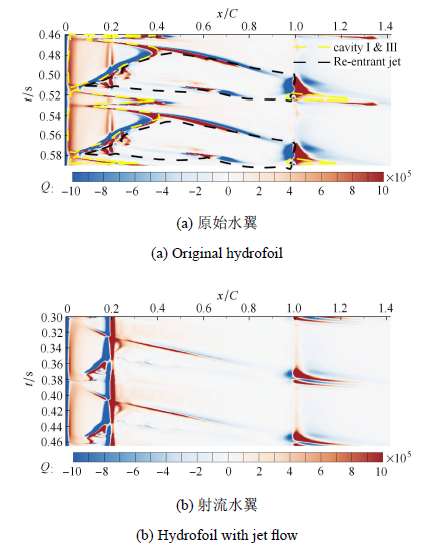Fig. 9   Spatiotemporal distribution contours of $Q$-criterion around NACA66 hydrofoil

## 3 结论

(1) 水翼尾缘游离型空穴溃灭时存在瞬间高压,并向水翼前缘传播;而采用主动射流后,该瞬间高压得到显著消减.

(2) 回射流区域的发展受限于较高的压力梯度. 采用射流后,水翼吸力面压力梯度减小,射流孔附近压力增高,回射流初生的时间延迟,回射流的强度降低,有效抑制了空化的脱落发展.

(3) 水翼空化的发展和流场中的涡结构有着紧密的联系. $Q$正值分布与水翼前缘附着型空穴和尾缘的游离型空穴形状相契合;$Q$负值分布与回射流的外部轮廓相契合. 说明前缘和尾缘空穴内部存在旋涡作用,而回射流对空穴也存在剪切作用.

(4) 射流对前缘附着型空穴和回射流具有剪切作用,抑制了回射流向前缘的推进和前缘附着型空穴的向后发展.

## 参考文献 原文顺序 文献年度倒序 文中引用次数倒序 被引期刊影响因子

( Pan Sensen, Peng Xiaoxing . Cavitation Mechanism. Beijing: National Defense Industry Press, 2013(in Chinese))

( Ji Bin, Cheng Huaiyu, Huang Biao , et al.

Research progresses and prospects of unsteady hydrodynamics characteristics for cavitation

( Huang Biao, Wang Guoyu, Wang Fufeng , et al.

Experimental investigation on flow field structure of unsteady cavitation flow

Journal of Experimental Mechanics, 2011,26(4):417-424(in Chinese))

( Gu Wei, He Yousheng, Hu Tianqun .

Noise feature of cavitation flows on axisymmetric bodies and transient characteristics of cavity interface

Journal of Shanghai Jiaotong University, 2000,34(8):1026-1030(in Chinese))

( Du Tezhuan, Wang Yiwei, Huang Chenguang , et al.

Study of coupling effects of underwater launched vehicle

Chinese Journal of Theoretical and Applied Mechanics, 2017,49(4):782-792(in Chinese))

( Yu Ting, Tian Zhong, Wang Wei , et al.

Characteristics of energy dissipation and cavitation for contracted plug in discharge tunnel

Shuili Xuebao, 2011,42(2):211-217(in Chinese))

( Wang Yiwei, Huang Chenguang .

Research progress on hydrodynamics of high speed vehicles in the underwater launching process

Callenaere M, Franc JP, Michel JM , et al.

The cavitation instability induced by the development of a re-entrant jet

Journal of Fluid Mechanics, 2001,444:223-256

Furness S, Hutton SP .

Experimental and theoretical studies of two-dimensional fixed-type cavities

ASME Journal of Fluids Engineering, 1975(4):515-521

Kawanami Y, Kato H, Yamaguchi H , et al.

Mechanism and control of cloud cavitation

ASME Journal of Fluids Engineering, 1997,119(4):788-794

Leroux JB, Astolfi JA, Billard JY .

An experimental study of unsteady partial cavitation

Journal of Fluids Engineering, 2004,126:94-101

Ganesh H, Mäkiharju SA, Ceccio SL .

Bubbly shock propagation as a mechanism for sheet-to-cloud transition of partial cavities

Journal of Fluid Mechanics, 2016,802:37-78

Wang C, Huang B, Wang G , et al.

Unsteady pressure fluctuation characteristics in the process of breakup and shedding of sheet/cloud cavitation

International Journal of Heat and Mass Transfer, 2017,114:769-785

( Wang Changchang, Wang Guoyu, Huang Biao .

Numerical simulation of shock wave dynamics in transient turbulent cavitating flows

Chinese Journal of Theoretical and Applied Mechanics, 2018,50(5):990-1002(in Chinese))

Tsujimoto Y .

Stability analysis of cavitating flows through inducers

// Fluid Dynamics of Cavitation and Cavitating Turbo Pumps,Vienna: Springer, 2007

Ji B, Luo XW, Roger EA , et al.

Large Eddy Simulation and theoretical investigations of the transient cavitating vortical flow structure around a NACA66 hydrofoil

International Journal of Multiphase Flow, 2015,68:121-134

Franc JP, Michel JM .

Fundamentals of cavitation

Fluid Mechanics & Its Applications, 2004,76(11):1-46

Gad-el-hak M . Flow Control: Passive, Active, and Reactive Flow Management. New York: Cambridge University Press, 2000

( Zhan Peiguo, Cheng Yahong, Zhao Xin .

A review of active flow control technology

Aeronautical Science & Technology, 2010(5):2-6(in Chinese))

[博士论文]. 大连:大连理工大学, 2013

( Wang Chuanzhou .

Cavity flow mechanism analysis and passive flow control technology research

[PhD Thesis]. Dalian: Dalian University of Technology, 2013(in Chinese))

Timoshevskiy MV, Zapryagaev II, Pervunin KS , et al.

Manipulating cavitation by a wall jet: Experiments on a 2D hydrofoil

International Journal of Multiphase Flow, 2018,99:312-328

Timoshevskiy MV, Churkin SA, Kravtsova AY , et al.

Cavitating flow around a scaled-down model of guide vanes of a high-pressure turbine

International Journal of Multiphase Flow, 2016,78:75-87

Lu SP, Wang W, Wang XF , et al.

Experiment research on cavitation control by active injection

// The 10th International Symposium on Cavitation 2018, May 14, 2018 to May 16, Baltimore, Maryland, USA

( Ji Bin, Bai Xiaorui, Zhu Ye , et al.

A review of the fundamental investigations of cavitation in hydraulic machinery

Chinese Journal of Hydrodynamics(series A), 2017,32(5):542-550(in Chinese))

Gopalan S, Katz J .

Flow structure and modeling issues in the closure region of attached cavitation

Physics of Fluids, 2000,12(4):895-911

( Zhang Jiayue, Li Daqin, Wu Qin , et al.

Numerical investigation on cavity structures and hyrodynamics of the vehicle during vertical water-entry

Chinese Journal of Theoretical and Applied Mechanics, 2019,51(3):803-812(in Chinese))

Yuan W, Sauer J, Schnerr GH .

Modeling and computation of unsteady cavitation flows in injection nozzles

Mécanique & Industries, 2001,2(5):383-394

Reboud JL, Stutz B, Coutier O .

Two phase flow structure of cavitation: experiment and modeling of unsteady efiects

// 3rd International Symposium on Cavitation, 1998

Wang W, Zhang QD, Tang T , et al.

Numerical study of the impact of water injection holes arrangement on cavitation flow control

Science Progress, (October 2019), doi: 10.1177/0036850419877742

Lu SP, Wang W, Wang XF , et al.

Experiment research on cavitation control by active injection

// The 10th International Symposium on Cavitation (CAV2018) 2018, May 14, 2018 to May 16, Baltimore, Maryland, USA

( Wang Wei, Xu Ruiduo, Yi Qi , et al.

Influence of re-entrant jet strength on cavitation characteristics of hydrofoil

Journal of Drainage and Irrigation Machinery Engineering, 2016,34(11):921-926,940(in Chinese))

( Wang Changchang, Wang Guoyu, Huang Biao , et al.

Experimental investigation of cavitation characteristics and dynamics in compressible turbulent cavitating flows

Chinese Journal of Theoretical and Applied Mechanics, 2019,51(5):1296-1309(in Chinese))

Huang B, Wang GY .

Experimental and numerical investigation of unsteady cavitating flows through a 2D hydrofoil

Science China Technological Sciences, 2011,54(7):1801-1812

Belahadji B, Franc P, Michel M .

Cavitation in the rotational structures of a turbulent wake

Journal of Fluid Mechanics, 1995,287:383-403

Arakeri VH, Acosta AJ .

Viscous effects in the inception of cavitation on axisymmetric bodies

Journal of Fluids Engineering, 1973,95(4):519-527

/

 〈〉